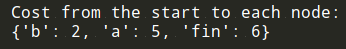# 《算法图解》学习笔记（七）：狄克斯特拉算法（附代码）

## 一、使用狄克斯特拉算法1. 找出“最便宜”的节点，即可在最短时间内到达的节点。
2. 更新该节点的邻居的开销，其含义将稍后介绍。
3. 重复这个过程，直到对图中的每个节点都这样做了。
4. 计算最终路径。• 前往节点A的更短路径（时间从6分钟缩短到5分钟）
• 前往终点的更短路径（时间从无穷大缩短到7分钟）• 前往节点B需要2分钟
• 前往节点A需要5分钟
• 前往终点需要6分钟1. 找出最便宜的节点，即可在最短时间内前往的节点。
2. 对于该节点的邻居，检查是否有前往它们的更短路径，如果有，就更新其开销。
3. 重复这个过程，直到对图中的每个节点都这样做了。
4. 计算最终路径。

## 二、术语## 三、换钢琴## 四、负权边## 五、实现graph = {}


graph["you"] = ["alice", "bob", "claire"]graph["start"] = {}
graph["start"]["a"] = 6
graph["start"]["b"] = 2>>> print graph["start"].keys()
["a", "b"]


>>> print graph["start"]["a"]
2
>>> print graph["start"]["b"]
6


graph["a"] = {}
graph["a"]["fin"] = 1
graph["b"] = {}
graph["b"]["a"] = 3
graph["b"]["fin"] = 5
graph["fin"] = {} # 终点没有任何邻居infinity = float("inf")


infinity = float("inf")
costs = {}
costs["a"] = 6
costs["b"] = 2
costs["fin"] = infinityparents = {}
parents["a"] = "start"
parents["b"] = "start"
parents["fin"] = None


processed = []# 在未处理的节点中找出开销最小的节点
node = find_lowest_cost_node(costs)
# 这个while循环在所有节点都被处理过后结束
while node is not None:
cost = costs[node]
neighbors = graph[node]
# 遍历当前节点的所有邻居
for n in neighbors.keys():
new_cost = cost + neighbors[n]
# 如果经当前节点前往该邻居更近，
if costs[n] > new_cost:
# 就更新该邻居的开销
costs[n] = new_cost
# 同时将该邻居的父节点设置为当前节点
parents[n] = node
# 将当前节点标记为处理过
processed.append(node)
# 找出接下来要处理的节点，并循环
node = find_lowest_cost_node(costs)def find_lowest_cost_node(costs):
lowest_cost = float("inf")
lowest_cost_node = None
for node in costs: # 遍历所有的节点
cost = costs[node]
if cost < lowest_cost and node not in processed: # 如果当前节点的开销更低且未处理过，
lowest_cost = cost # 就将其视为开销最低的节点
lowest_cost_node = node
return lowest_cost_node


python版本的代码如下：

# the graph 绘制图
graph = {}
graph["start"] = {}
graph["start"]["a"] = 6
graph["start"]["b"] = 2

graph["a"] = {}
graph["a"]["fin"] = 1

graph["b"] = {}
graph["b"]["a"] = 3
graph["b"]["fin"] = 5

graph["fin"] = {}

# the costs table 成本表
infinity = float("inf")
costs = {}
costs["a"] = 6
costs["b"] = 2
costs["fin"] = infinity

# the parents table 父节点表
parents = {}
parents["a"] = "start"
parents["b"] = "start"
parents["fin"] = None

processed = []

def find_lowest_cost_node(costs):
lowest_cost = float("inf")
lowest_cost_node = None
# 遍历每个节点。
for node in costs:
cost = costs[node]
# 如果这是目前为止最低的成本而且还没有被处理......
if cost < lowest_cost and node not in processed:
# ......设置为新的最低成本节点。
lowest_cost = cost
lowest_cost_node = node
return lowest_cost_node

# 查找尚未处理的最低成本节点。
node = find_lowest_cost_node(costs)
# 如果已经处理了所有节点，那么while循环就完成了。
while node is not None:
cost = costs[node]
# 遍历此节点的所有邻居。
neighbors = graph[node]
for n in neighbors.keys():
new_cost = cost + neighbors[n]
# 如果通过这个节点到这个邻居比较便宜的话......
if costs[n] > new_cost:
# ......更新此节点的成本。
costs[n] = new_cost
# 此节点将成为此邻居的新父节点。
parents[n] = node
# 将节点标记为已处理。
processed.append(node)
# 找到下一个要处理的节点，然后循环。
node = find_lowest_cost_node(costs)

print("Cost from the start to each node:")
print(costs)## 六、总结

• 广度优先搜索用于在非加权图中查找最短路径。
• 狄克斯特拉算法用于在加权图中查找最短路径。
• 仅当权重为正时狄克斯特拉算法才管用。
• 如果图中包含负权边，请使用贝尔曼福德算法。

## 参考文章

• 《算法图解》